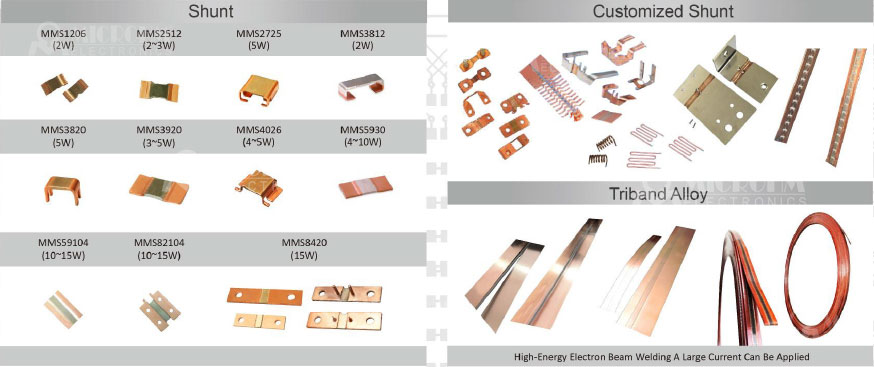Tel:400-803-9333
• Welcome to Microhm

#Position：Home » Company News

# Shunt Resistors for Current Sense

Writer：Microhm Page View：Date：2018-06-11

Ammeters are used to measure the AC or DC by measuring the voltage drop over a precision resistor with a known resistance. The current flow is calculated by using Ohm’s law.

Most ammeters have an built-in resistor to measure the current. However, when the current is too high for the ammeter, the ammeter is placed in parallel with an accurate shunt resistor - ammeter shunt which is a high precision manganin resistor with a low resistance value. The current is divided over the shunt and the ammeter, so only a small percentage flows through the ammeter.  In this way, large currents can still be measured. By correctly scaling the ammeter, the actual amperage can be directly measured. Using this configuration, in theory the maximum amperage that can be measured is endless. However, the voltage rating of the measurement device must not be exceeded. This means that the maximum current multiplied by the resistance value, cannot be higher than the voltage rating. Also, the resistance value should be as low as possible to limit the interference with the circuit.Shunt resistors have a maximum current rating. The resistance value is given by the voltage drop at the maximum current rating. For example, a shunt resistor rated with 100A and 50mV has a resistance of 50 / 100 = 0.5 mOhm. Other important parameters include the resistance tolerance, the temperature coefficient of resistance and the power rating.

It is important to carefully choose the position of the shunt in the circuit. When the circuit shares a common ground with the measurement device, often is chosen to place the shunt as close to the ground as possible.

Keywords：